Entropy Bed Sizes Quilt Pattern Now Available! Sign up for the newsletter to recieve a special discount code!

### Let's break down an Entropy Block first

An Entropy block requires12 half square triangles and 4 background squares.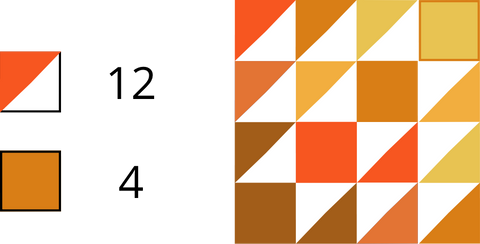There are three options to building an entropy block, Super scrappy, normal, or inverse. Each option will be described below.

## Super Scrappy

Uses scraps and mismatched fabrics for all units. You will base the number of blocks you can make on the amount of scraps you have instead of using additional yardage.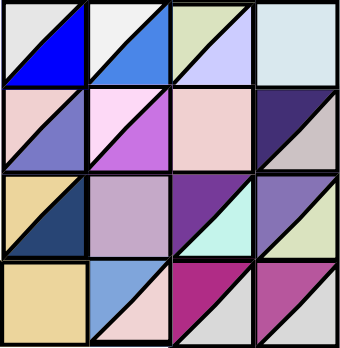## Normal and Inverse Entropy Block

You can choose to do a "normal" entropy quilt block seen below, to the left, where the half square triangle color is oriented inwards, and the background squares are a single color oriented outward. The other option is what will be referred to as an "inverse" entropy quilt block, where the color that contrasts more is facing outward and the background squares are colored.# Cutting

So you've already sorted out the usable scraps or you've picked the precuts you want to use and  now you are ready to cut your fabrics into usable squares. (Continue to scroll to Precuts if that is what you are using)

## Using Scraps?

Start by working one container at a time. First, start with your scraps you will be cutting down to 5" squares, then 7.25" squares and then your 10" squares.  While cutting these scraps, accuracy in cutting the squares will help ensure the units come out to the correct size of 4.5" squares when sewn together and cut.

You can use a large 12.5" x12.5" ruler to cut all of the required squares. One way I like to improve my accuracy as well as cut down on my cutting time is by marking my ruler with Washi Tape like below.If you look closely at the picture below, you can see that I put the tape on the outside of the line that marks 5". This helps ensure that I get a full 5" square with a scant extra when ever I am cutting to size. I recommend doing the same when cutting the 7.25" and 10" squares.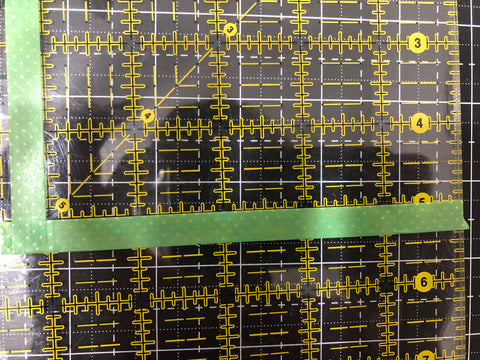Check out this video to see how I trim them one at a time.

If you have a rotating cutting mat, you can stack and whack scraps accurately by doing the following!

## So you've finished cutting?

Now you will need to determine the amount of squares you have of each size (4.5",5",7.25", & 10"). I suggest writing these numbers down. If you haven't already decided, you will need to determined if you will make "Super scrappy", "normal", or "inverse" entropy blocks. The normal and inverse use additional yardage to create half square triangles while the super scrappy pairs scraps together. For each half square method, we need two squares of the same size, regardless of method.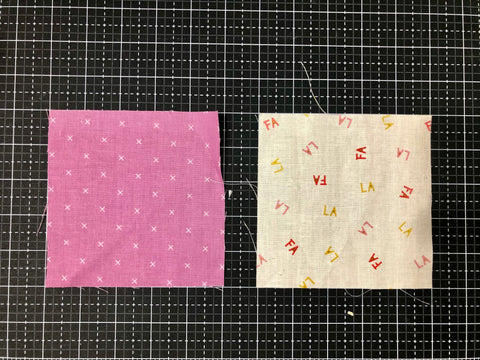## Super Scrappy Version

To determine how many half squares triangles you can make by pairing the scrap squares together, count the amount of 5" squares, 7.25" squares, and 10" squares.

Use the following equation, and be sure to round down to the nearest even number before hand. So if I have (67) 5" squares, you will round down to (66) 5" squares.

In the equation,  x=the amount of 5" squares you have, y= the amount of 7.25" squares and z=the amount of 10" squares you have to determine how many.

(x)+(y2)+(z*4)=Amount of HSTs

To find out how many blocks you can make divide the amount of HSTs by 12.

HSTs/12=blocks

Then to find out how many 4.5" squares you need, multiply the amount of blocks by 4.

blocks x 4= amount of 4.5" squares.

For example, if I have (62) 5" squares, (7) 7.25" squares, & (6) 10" squares I would round the (7) 7.25" squares down to (6) and I would have a total of (62)+(6*2)+(6*4)= 98 HSTs

To find how many blocks I can make with that amount of HSTs,  I divide 98/12=8.1, rounded down =8 blocks.

For 8 blocks, I need (4) 4.5" squares per block, so 8*4= 32

I need (32) 4.5" squares for my 8 blocks.

Use the excel spreadsheet and input your numbers in the cells marked yellow to have the worksheet output the above numbers for you. Click Here.

## Scraps & Yardage

If you will be using yardage instead of scraps, you will still need to know how many HSTs you can make from your scraps, but the equation will be as following:

(x2)+(y4)+(z*8)=Amount of HSTs

To find out how many blocks you can make divide the total amount of HSTs by 12.

HSTs/12=blocks

Then to find out how many 4.5" squares you need, multiply the amount of blocks by 4.

blocks x 4= amount of 4.5" squares.

To determine how much fabric you will need to supplement for yardage you can use the following equation. It assumes you are using 42" WOF.

x=5" squares, y=7.25" squares, z=10" squares, & f=4.5 squares

Normal Entropy Block yardage calculation

((x/8)*5)+((y/5)*7.25)+((z/4)*10)+((f/9)*4.5)= y

You can use the included excel spread sheet to calculate yardage for you. Just fill in the cells marked in yellow. Click here.

Inverse Entropy Block Yardage Calculation

((x/8)*5)+((y/5)*7.25)+((z/4)*10)= y

Now that you know how many HSTs you have and how much additional yardage you will need. You can start planning your quilt layout with the following coloring sheet or you can wait until you have made your HSTs.

# Using Precuts

If you are using precuts, you need to decide; what your cutting plan is.

## Charm Packs (5") & Layer Cakes (10")

The charm packs (5") are easy and you can use them for 2-at-a-time HST construction, no extra cutting necessary. The same can be said for 10" layer cakes, which will be used for 8-at-a-time half square triangles. Now for fat eighths and fat quarters you will need to cut them down into 5", 7.25" and 10" sub-cuts.

## Fat Eighths (9"x22")

Below is the suggested way to cut a fat eighth; which measures 9"x22".As you can see above, you can get (3) 7.25" squares out of a fat eighth, which translates into the possibility of 12 HSTs if you pair them with additional yardage.

## Fat Quarters (18"x22")

Since Fat quarters are larger cuts, we are able to get a lot more sub-cuts out of them. You can choose to use as little or as much of each fat quarter as you like. Here are some cutting suggestions. Also if you prefer 2-at-a-time HSTs instead of 8-at-a-time, you can substitute any 10" square for (4) 5" squares to make the same amount of half square triangles.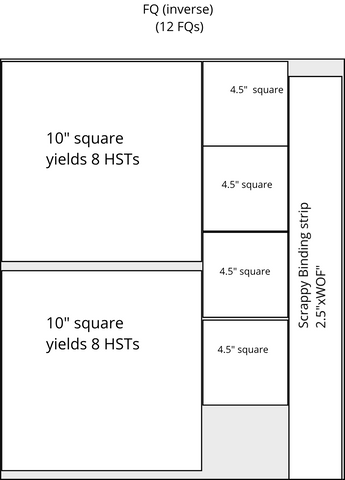At the top, you can see that it is labeled FQ Inverse and uses 12 FQs  to make a throw size quilt. This assumes that you pair each 10" square from the fat quarters with background fabric. You would need 1 3/4 yards of additional fabric to cut (24) 10" background squares and 16 additional 4.5" colored squares.At the top, you can see that it is labeled FQ normal and  16 FQs are needed to make a throw size quilt. This assumes that you pair each 10" square from the fat quarters with background fabric. You would need 3 yards of additional fabric to make this version and would cut (64) 4.5" squares, (16) 10" squares and (32) 5" squares from the additional yardage.At the top, you can see that it is labeled FQ normal and  8 FQs are needed to make a throw size quilt. This assumes that you pair each 10" square from the fat quarters with background fabric. You would need 3 yards of additional fabric to make this version and would cut from the background fabric (16) 10" squares, (32) 5" squares and  (64) 4.5" squares.